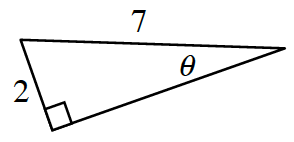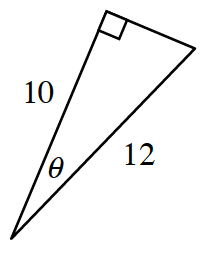### Home > PC3 > Chapter 1 > Lesson 1.3.2 > Problem1-125

1-125.

Solve for $θ$ in each triangle below.

1.$\sin(\theta)=\frac{2}{7}$

1.$\cos(\theta)=\frac{10}{12}$